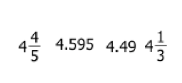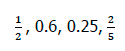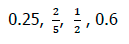Ratios
Equivalencies
Compare and Order
GCF and
Simplifying
Fractions
Exponents
100

There are 10 white marbles and 3 blue marbles. What is the ratio of white to blue marbles?

What is 10:3?

100

Decimals to Percents .49What is 49%

100

5/8 is equivalent to what decimal?

What is 0.625?

100

Simplify 15/36.

What is 5/12?

100

This is what fractions must have in order to add or subtract them.

What is a common denominator?

100

Simplify

32 = ___

What is 9?

200

How many ways can a ratio be written?

What is three?

200

Fractions to Percents 2/5

What is 40%?

200

1/8 is equivalent to what decimal?

What is 0.125?

200

The Greatest Common Factor of 20 and 30 is what?

What is 10?

200

1 2/3 + 2 3/4

What is 4 5/12

200

70

What is one?

300

How to determine if two ratios are equivalent?

What is cross multiply?

300

Decimals to Fractions .72

What is 72/100?

300What is 0.6?

300

Simplify 17/21.

What is 17/21?

300

What is 3 4/5 as an improper fraction?

What is 19/5?

300

What number is a perfect square between 49 and 81?

What is 64?

400

The ratio of boys to girls is 1:2, if there's 12 boys how many girls are there?What is 24?

400

Percents to Fractions 56%

What is 56/100?

400

Arrange from Greatest to Least.What is?

400

This is the first step to simplifying a fraction.

What is find the GCF of the numerator and denominator?

400

2/3 divided by 1/6

What is 4?

400

A Factor that is being repeated in the Multiplication sentence.What is the base?

500

Write the following ratio simplified : 44 / 11

What is 4/1?

500

Decimals to Fractions 0.2

What is 2/10 or 1/5?

500

List these numbers in order from least to greatest.What is?

500

Simplify.

77/21What is 11/3?

500

3/4 x 2/3What is 1/2?

500

106

What is 1,000,000?

Click to zoom